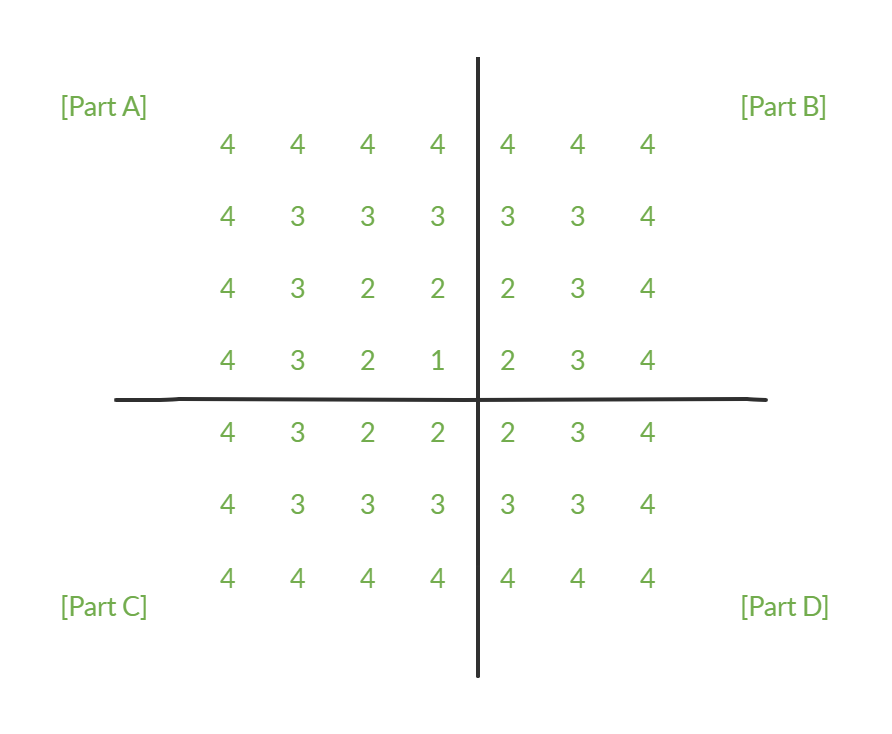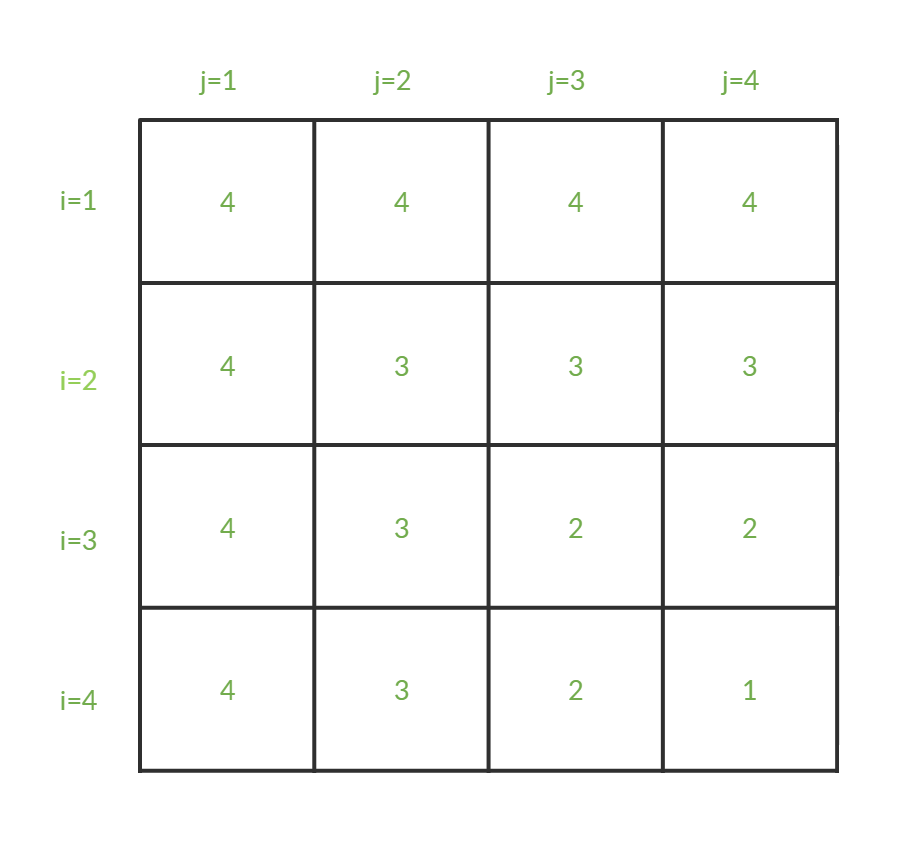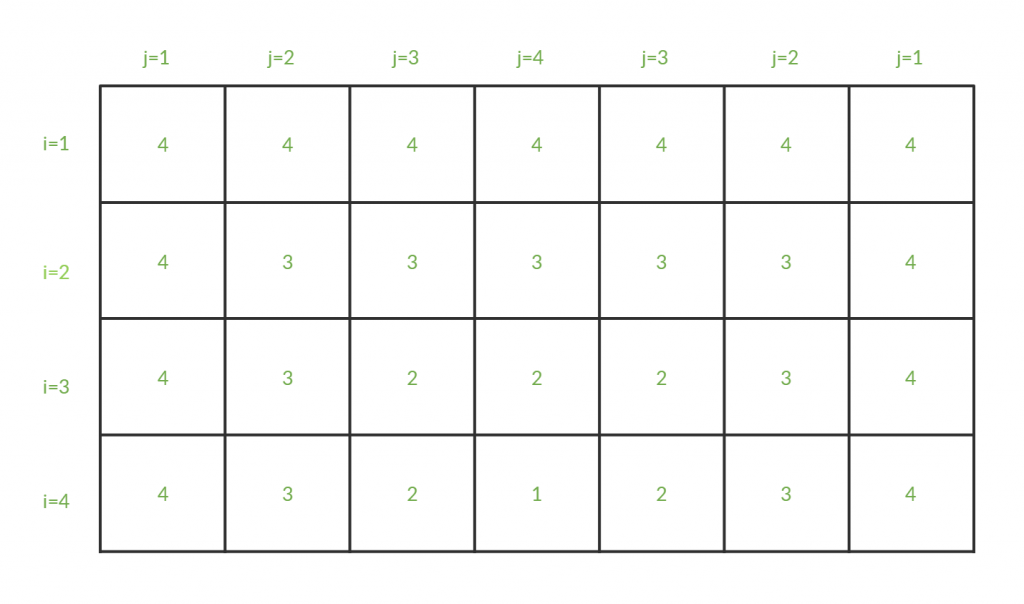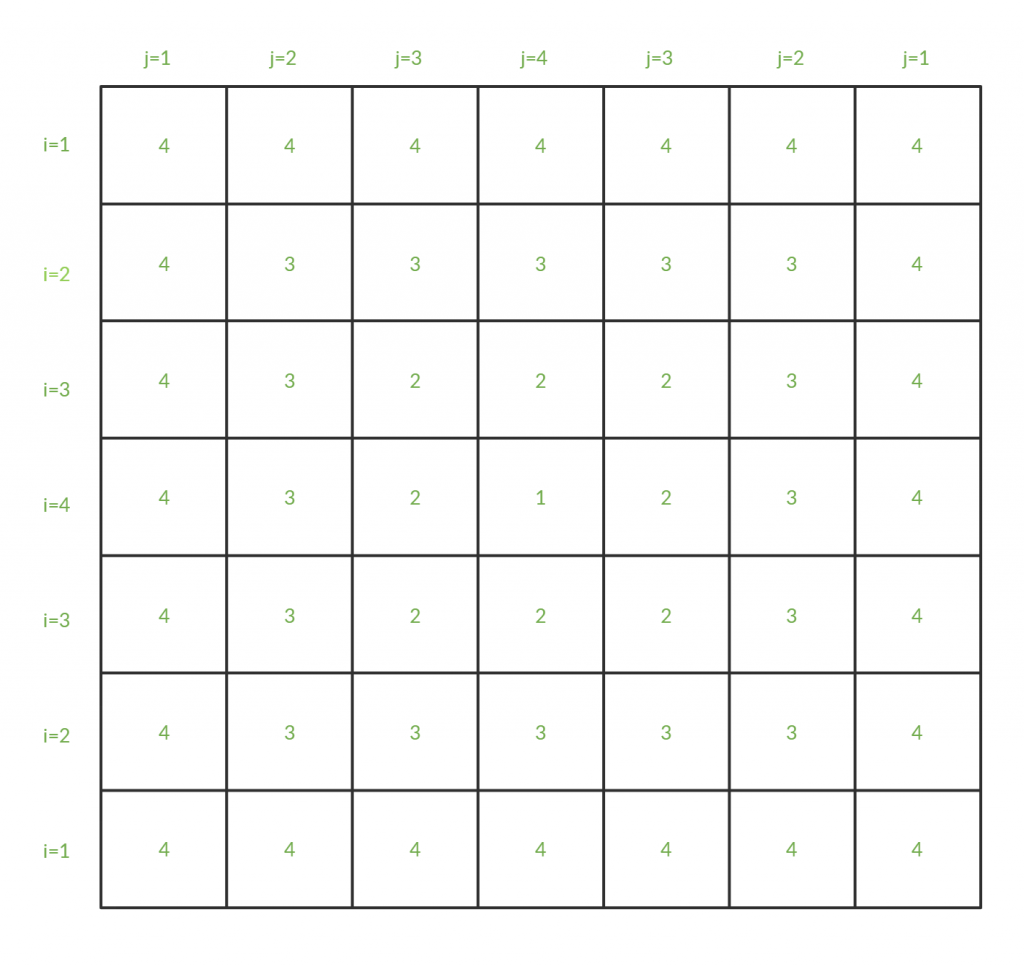# How to learn Pattern printing easily?

• Difficulty Level : Medium
• Last Updated : 24 Nov, 2021

The task is to print a pattern as shown in the example for a given integer value.
The goal is not to print just this one pattern, it is to learn the best approach to solve this kind of problems as these questions are frequently asked in coding exams and in job interviews.
Examples:

```Input: N = 4
Output:
4 4 4 4 4 4 4
4 3 3 3 3 3 4
4 3 2 2 2 3 4
4 3 2 1 2 3 4
4 3 2 2 2 3 4
4 3 3 3 3 3 4
4 4 4 4 4 4 4

Input: N = 7
Output:
7 7 7 7 7 7 7 7 7 7 7 7 7
7 6 6 6 6 6 6 6 6 6 6 6 7
7 6 5 5 5 5 5 5 5 5 5 6 7
7 6 5 4 4 4 4 4 4 4 5 6 7
7 6 5 4 3 3 3 3 3 4 5 6 7
7 6 5 4 3 2 2 2 3 4 5 6 7
7 6 5 4 3 2 1 2 3 4 5 6 7
7 6 5 4 3 2 2 2 3 4 5 6 7
7 6 5 4 3 3 3 3 3 4 5 6 7
7 6 5 4 4 4 4 4 4 4 5 6 7
7 6 5 5 5 5 5 5 5 5 5 6 7
7 6 6 6 6 6 6 6 6 6 6 6 7
7 7 7 7 7 7 7 7 7 7 7 7 7```

For the tutorial, an example of N = 4 is used.

• Step 1: First of all, analyse the pattern for any lines of symmetry. Here our pattern is both vertically and horizontally symmetrical, so draw the lines of symmetry like this,• After breaking the pattern in parts, first try to draw only the upper-left part, namely, part A. If there is not any line of symmetry, jump to Step 2.
• Step 2: Now associate each cell i.e. element with a row and column no.( usually denoted by i and j, respectively) Just like,• From now on, a cell is denoted by C(i, j) with its row and column no.
• Step 3: In this step, try and find a relation between the value of C(i, j) with i and/or j. Usually, the value of C depends on the value of N and values of i and j relative to each other. To elaborate,
– In 1st row, every element is same i.e. 4(=N). So it is not of much help.
– In 2nd row, C can be seen decreasing from of 4 to 3 for i>=j and then it stays 3 for next i=j and then it stays 2 for next i=j for all values of j, C can be seen decreasing from 4 to 1.
So in every row, C starts from N, decreases by 1 until i>=j, and then becomes constant. Here the value of C depends upon the smaller between i and j and the formula could be:

`C(i, j) = N - min(i, j) + 1`
• So our approach should be:

## C++

 `#include ``using` `namespace` `std;`` ` `int` `main()``{``    ``int` `N = 4, i, j, min;``    ``cout << ``"Value of N: "` `<< N << endl;`` ` `    ``for` `(i = 1; i <= N; i++) {``        ``for` `(j = 1; j <= N; j++) {``            ``min = i < j ? i : j;``            ``cout << N - min + 1;``        ``}``        ``cout << endl;``    ``}``    ``return` `0;``}`

## Java

 `// Java program to find triplets ``// a[i]>a[j]>a[k] and i

## Python3

 `# Python3 program to find triplets ``# a[i]>a[j]>a[k] and i

## C#

 `// C# program to find triplets ``// a[i]>a[j]>a[k] and i

## Javascript

 ``

•
Output:

```Value of N: 4
4444
4333
4322
4321```

• If the pattern didn’t have any symmetry and is now completed, the work is done. But for patterns with symmetry, they are still incomplete and it is required to go to step 4.

• Step 4: Now include part B of the pattern in the picture and associate those elements with column numbers. But don’t assign column numbers serial-wise, instead, assign them the same column number that was assigned to its mirror column in part A.•
• Step 5: Now change the code to append part B. To do that, it is just needed to rerun the inner loop for j = n-1 to j=1.

## C++

 `#include ``using` `namespace` `std;`` ` `int` `main()``{``    ``int` `N = 4, i, j, min;``    ``cout << ``"Value of N: "` `<< N << endl;`` ` `    ``for` `(i = 1; i <= N; i++) {``        ``for` `(j = 1; j <= N; j++) {``            ``min = i < j ? i : j;``            ``cout << N - min + 1;``        ``}``        ``for` `(j = N - 1; j >= 1; j--) {``            ``min = i < j ? i : j;``            ``cout << N - min + 1;``        ``}``        ``cout << endl;``    ``}``    ``return` `0;``}`

## Java

 `class` `GFG ``{``    ``public` `static` `void` `main(String[] args) ``    ``{``        ``int` `N = ``4``, i, j, min;``        ``System.out.println(``"Value of N: "` `+ N);``     ` `        ``for` `(i = ``1``; i <= N; i++) ``        ``{``            ``for` `(j = ``1``; j <= N; j++) ``            ``{``                ``min = i < j ? i : j;``                ``System.out.print(N - min + ``1``);``            ``}``            ``for` `(j = N - ``1``; j >= ``1``; j--)``            ``{``                ``min = i < j ? i : j;``                ``System.out.print(N - min + ``1``);``            ``}``            ``System.out.println();``        ``}``    ``}``}`` ` `// This code is contributed by PrinciRaj1992`

## Python3

 `N ``=` `4``;``print``(``"Value of N: "``, N);`` ` `for` `i ``in` `range``(``1``, N ``+` `1``):``    ``for` `j ``in` `range``(``1``, N ``+` `1``):``        ``min` `=` `i ``if` `i < j ``else` `j;``        ``print``(N ``-` `min` `+` `1``, end ``=` `"");`` ` `    ``for` `j ``in` `range``(N ``-` `1``, ``0``, ``-``1``):``        ``min` `=` `i ``if` `i < j ``else` `j;``        ``print``(N ``-` `min` `+` `1``, end ``=` `"");``    ``print``();`` ` `# This code is contributed by Rajput-Ji`

## C#

 `using` `System;`` ` `class` `GFG ``{``    ``public` `static` `void` `Main(String[] args) ``    ``{``        ``int` `N = 4, i, j, min;``        ``Console.WriteLine(``"Value of N: "` `+ N);``     ` `        ``for` `(i = 1; i <= N; i++) ``        ``{``            ``for` `(j = 1; j <= N; j++) ``            ``{``                ``min = i < j ? i : j;``                ``Console.Write(N - min + 1);``            ``}``            ``for` `(j = N - 1; j >= 1; j--)``            ``{``                ``min = i < j ? i : j;``                ``Console.Write(N - min + 1);``            ``}``            ``Console.WriteLine();``        ``}``    ``}``}`` ` `// This code is contributed by Rajput-Ji`

## Javascript

 ``

•
Output:

```Value of N: 4
4444444
4333334
4322234
4321234```

•
• Step 6: Now includes part C and D of the pattern in the picture and associate those elements with row numbers in the same way the column numbers are assigned in step 4.•
• Step 7: Now, In the same way, as done in step 5, change the code to append part C and D. To do that, one just need to rerun the outer loop for i = n-1 to i=1.

## C++

 `#include ``using` `namespace` `std;`` ` `int` `main()``{``    ``int` `N = 4, i, j, min;``    ``cout << ``"Value of N: "` `<< N << endl;`` ` `    ``for` `(i = 1; i <= N; i++) {``        ``for` `(j = 1; j <= N; j++) {``            ``min = i < j ? i : j;``            ``cout << N - min + 1;``        ``}``        ``for` `(j = N - 1; j >= 1; j--) {``            ``min = i < j ? i : j;``            ``cout << N - min + 1;``        ``}``        ``cout << endl;``    ``}``    ``for` `(i = N - 1; i >= 1; i--) {``        ``for` `(j = 1; j <= N; j++) {``            ``min = i < j ? i : j;``            ``cout << N - min + 1;``        ``}``        ``for` `(j = N - 1; j >= 1; j--) {``            ``min = i < j ? i : j;``            ``cout << N - min + 1;``        ``}``        ``cout << endl;``    ``}``    ``return` `0;``}`

## Java

 `// Java implementation of the approach``class` `GFG ``{``public` `static` `void` `main(String[] args) ``{``    ``int` `N = ``4``, i, j, min;``    ``System.out.println(``"Value of N: "` `+ N);`` ` `    ``for` `(i = ``1``; i <= N; i++) ``    ``{``        ``for` `(j = ``1``; j <= N; j++)``        ``{``            ``min = i < j ? i : j;``            ``System.out.print(N - min + ``1``);``        ``}``        ``for` `(j = N - ``1``; j >= ``1``; j--)``        ``{``            ``min = i < j ? i : j;``            ``System.out.print(N - min + ``1``);``        ``}``        ``System.out.println();``    ``}``     ` `    ``for` `(i = N - ``1``; i >= ``1``; i--) ``    ``{``        ``for` `(j = ``1``; j <= N; j++) ``        ``{``            ``min = i < j ? i : j;``            ``System.out.print(N - min + ``1``);``        ``}``        ``for` `(j = N - ``1``; j >= ``1``; j--) ``        ``{``            ``min = i < j ? i : j;``            ``System.out.print(N - min + ``1``);``        ``}``        ``System.out.println();``    ``}``    ``}``}`` ` `// This code is contributed by 29AjayKumar`

## Python3

 `# Python3 implementation of the approach``N ``=` `4``print``(``"Value of N: "``, N) `` ` `for` `i ``in` `range``(``1``, N ``+` `1``): ``    ``for` `j ``in` `range``(``1``, N ``+` `1``): ``        ``min` `=` `i ``if` `i < j ``else` `j ``        ``print``(N ``-` `min` `+` `1``, end ``=` `" "``) `` ` `    ``for` `j ``in` `range``(N ``-` `1``, ``0``, ``-``1``): ``        ``min` `=` `i ``if` `i < j ``else` `j ``        ``print``(N ``-` `min` `+` `1``, end ``=` `" "``)`` ` `    ``print``()``     ` `for` `i ``in` `range``(N ``-` `1``, ``0``, ``-``1``): ``    ``for` `j ``in` `range``(``1``, N ``+` `1``): ``        ``min` `=` `i ``if` `i < j ``else` `j ``        ``print``(N ``-` `min` `+` `1``, end ``=` `" "``) `` ` `    ``for` `j ``in` `range``(N ``-` `1``, ``0``, ``-``1``): ``        ``min` `=` `i ``if` `i < j ``else` `j ``        ``print``(N ``-` `min` `+` `1``, end ``=` `" "``)`` ` `    ``print``()``     ` `# This code is contributed by sai prakash`

## C#

 `// C# implementation of the approach``using` `System;`` ` `class` `GFG ``{``public` `static` `void` `Main(String[] args) ``{``    ``int` `N = 4, i, j, min;``    ``Console.WriteLine(``"Value of N: "` `+ N);`` ` `    ``for` `(i = 1; i <= N; i++) ``    ``{``        ``for` `(j = 1; j <= N; j++)``        ``{``            ``min = i < j ? i : j;``            ``Console.Write(N - min + 1);``        ``}``        ``for` `(j = N - 1; j >= 1; j--)``        ``{``            ``min = i < j ? i : j;``            ``Console.Write(N - min + 1);``        ``}``        ``Console.WriteLine();``    ``}``     ` `    ``for` `(i = N - 1; i >= 1; i--) ``    ``{``        ``for` `(j = 1; j <= N; j++) ``        ``{``            ``min = i < j ? i : j;``            ``Console.Write(N - min + 1);``        ``}``        ``for` `(j = N - 1; j >= 1; j--) ``        ``{``            ``min = i < j ? i : j;``            ``Console.Write(N - min + 1);``        ``}``        ``Console.WriteLine();``    ``}``    ``}``}`` ` `// This code is contributed by Rajput-Ji`

## Javascript

 ``

Output:

```Value of N: 4
4444444
4333334
4322234
4321234
4322234
4333334
4444444```